# Relational Triple Extraction: One Step is Enough

## Basic Idea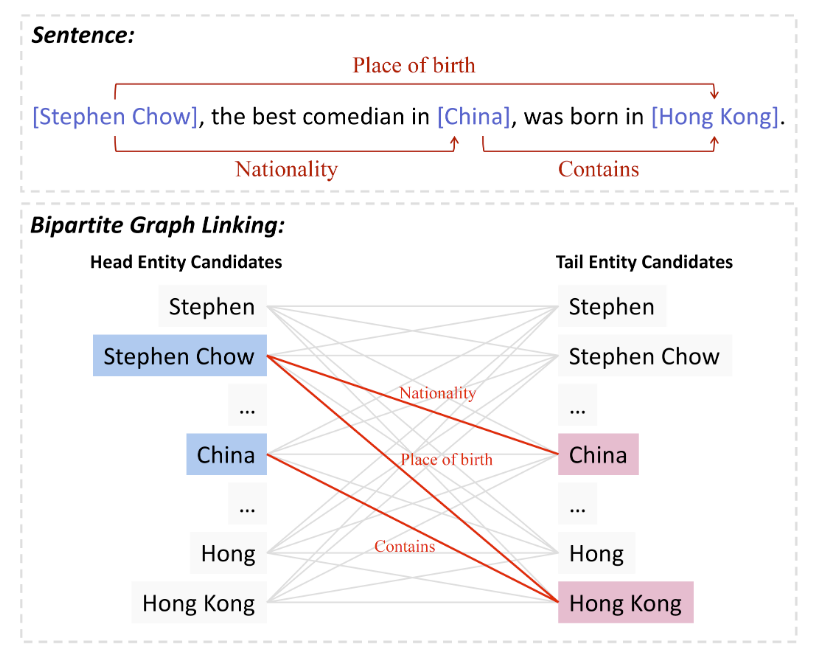## DirectRel

DirectRel的模型设计是十分简单的: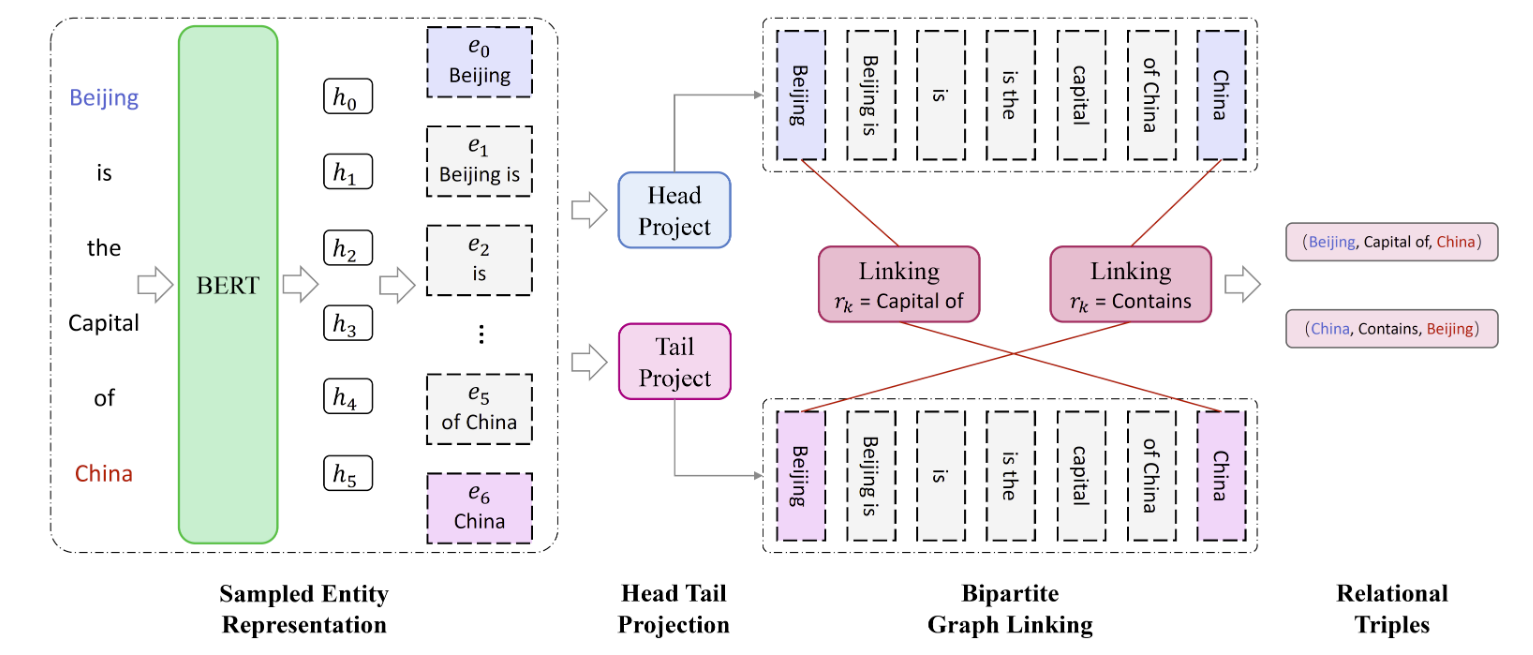### Candidate Entities Generation

$$|\mathcal{E}|=L \times C+\frac{C}{2}-\frac{C^2}{2}$$

$$\left[\boldsymbol{h}_1, \boldsymbol{h}_2, \ldots, \boldsymbol{h}_L\right]=B E R T\left(\left[\boldsymbol{x}_1, \boldsymbol{x}_2, \ldots, \boldsymbol{x}_L\right]\right)$$

$$\boldsymbol{e}_i=\frac{\boldsymbol{h}^{\text {start }}+\boldsymbol{h}^{e n d}}{2}$$

\begin{aligned} \boldsymbol{E}_{\text {head }} &= \boldsymbol{W}^{T}_{h}\boldsymbol{E}+ \boldsymbol{b}_{h}\\ \boldsymbol{E}_{\text {tail }} &= \boldsymbol{W}^{T}_{t}\boldsymbol{E}+ \boldsymbol{b}_{t} \end{aligned}

$$\boldsymbol{P}^k=\sigma\left(\boldsymbol{E}_{\text {head }}^T \boldsymbol{U}_k \boldsymbol{E}_{\text {tail }}\right)$$

### Objective Function

$$\mathcal{L}=-\frac{1}{|\overline{\mathcal{E}}| \times K \times|\overline{\mathcal{E}}|} \times \sum_{i=1}^{|\overline{\mathcal{E}}|} \sum_{k=1}^K \sum_{j=1}^{|\overline{\mathcal{E}}|}\left(y_t \log \left(\boldsymbol{P}_{i j}^k\right)+\left(1-y_t\right) \log \left(1-\boldsymbol{P}_{i j}^k\right)\right)$$

## Experiments

### Datasets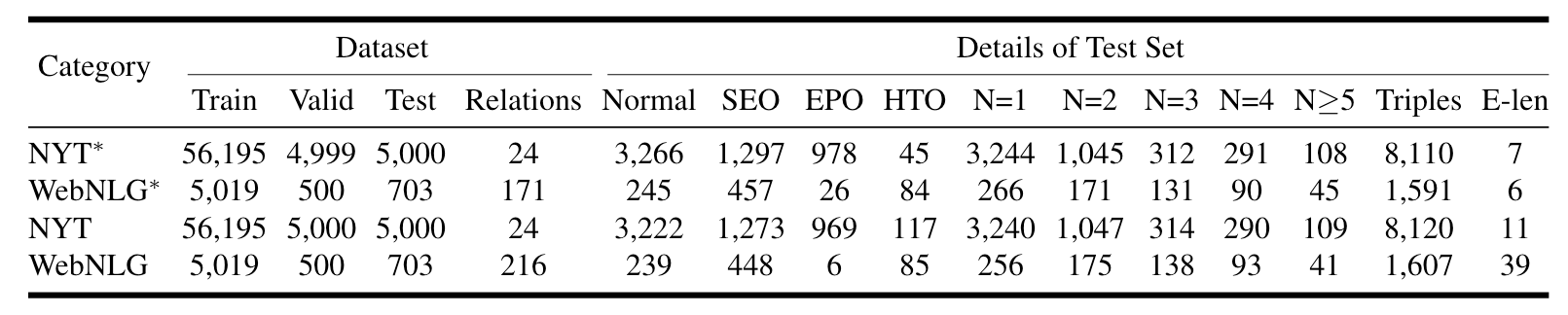### Main Results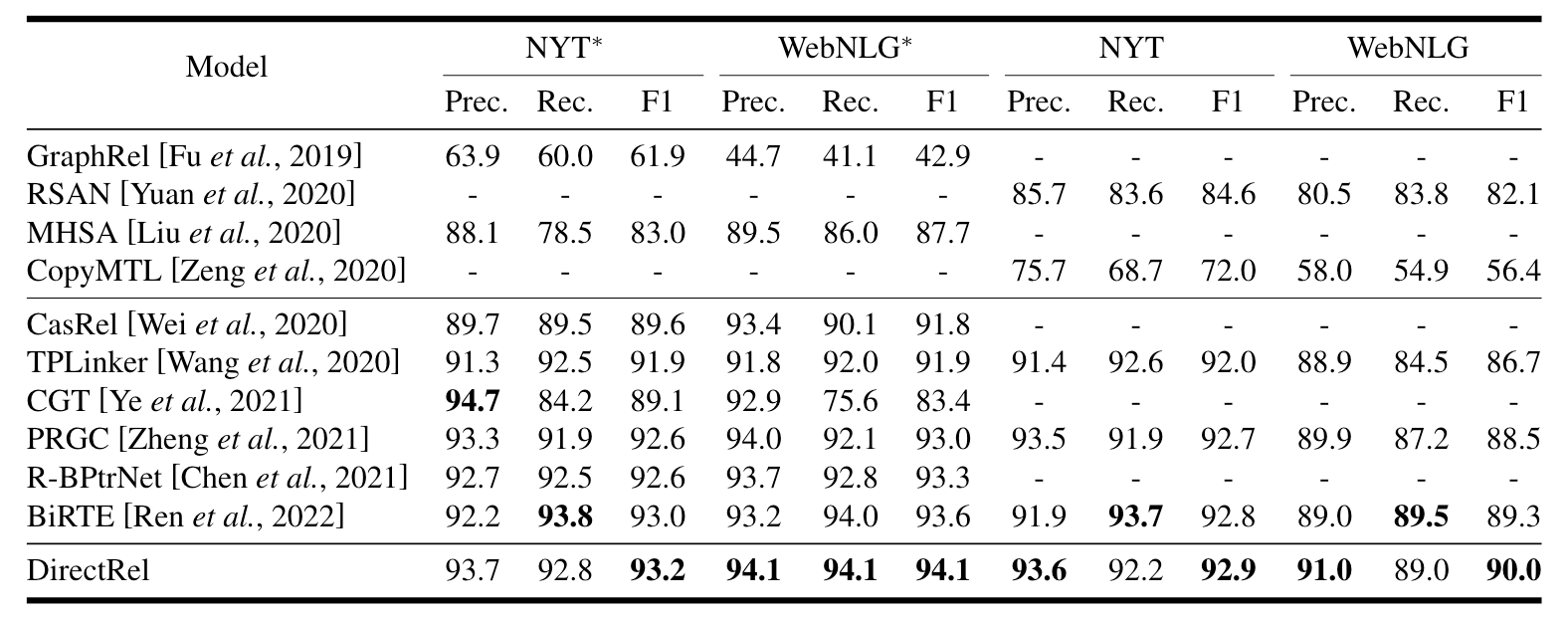DirectRel在Baseline里面达到了SOTA, 在NYT上领先不是很多.

### Detailed Results on Complex Scenarios

DirectRel在不同类型三元组的表现如下: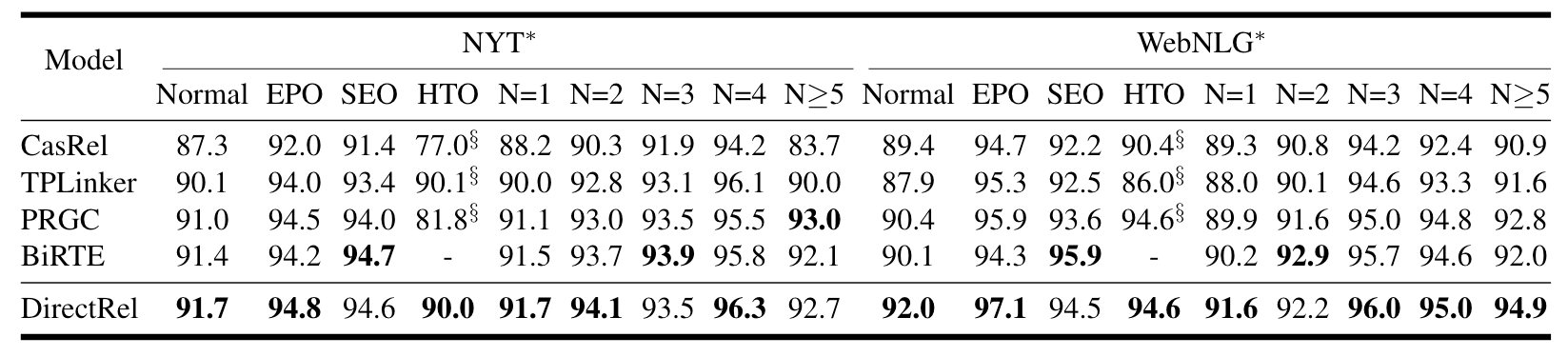DirectRel似乎对SEO的处理要略差一点, 说明DirectRel处理一对多的三元组能力差一点. 它其他的都还不错.

### Results on Different Sub - tasks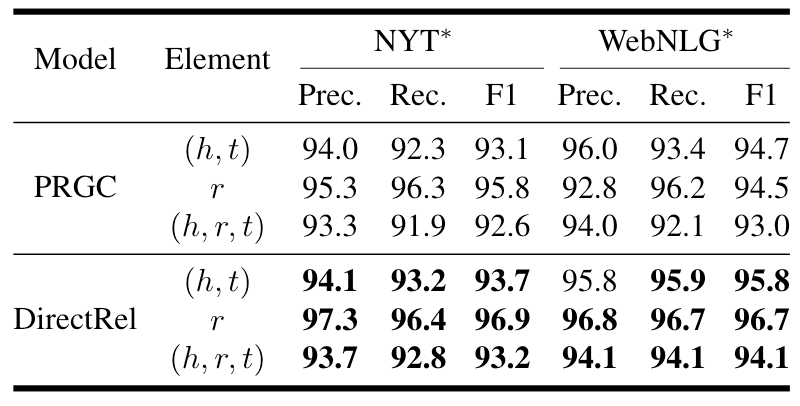### Parameter Analysis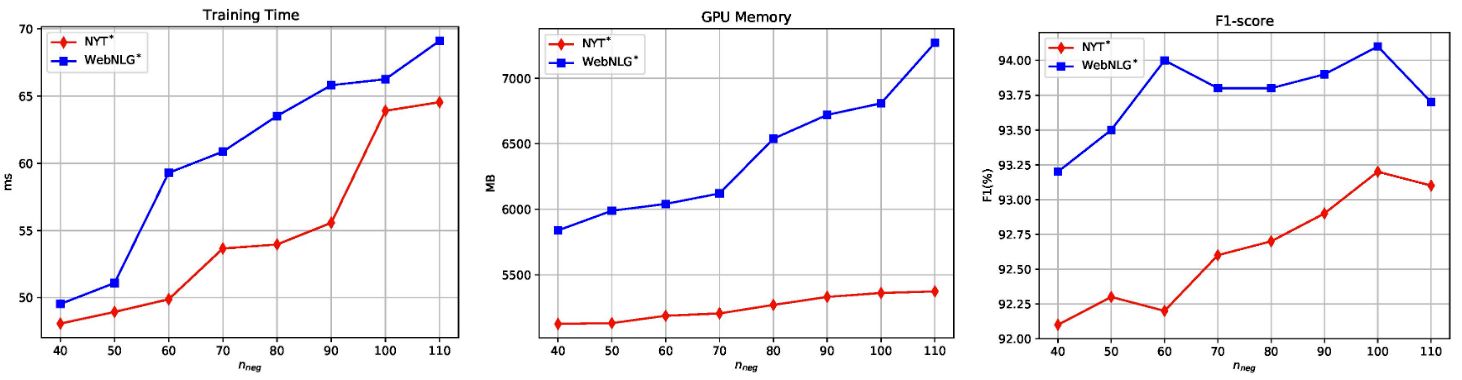### Error Analysis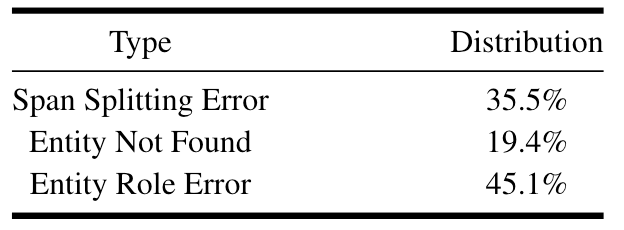## Summary

上一篇UniRE: A Unified Label Space for Entity Relation Extraction
UniRE: A Unified Label Space for Entity Relation Extraction本文是论文UniRE: A Unified Label Space for Entity Relation Extract
2022-09-22OneRel: Joint Entity and Relation Extraction with One Module in One Step

2022-08-14
目录